# 基于Hdl Coder实现卡尔曼滤波算法

下面以卡尔曼滤波为例，具体讲述如何基于Hdl Coder实现卡尔曼滤波算法实现。首先简单介绍下卡尔曼滤波算法：

Kalman filtering的经典五方程，在进行卡尔曼滤波的程序设计前必须要充分理解这五个方程的定义、推导以及相关变量的设定。

(1) 对于现在状态的预测方程：

X(k|k-1)=A X(k-1|k-1)+B U(k) ……….. (1)

P(k|k-1)=A P(k-1|k-1) A’+Q ……… (2)

(2)对于现在状态的更新方程：（拿过去的真实值得到现在的预测值，再集合现在的测量值Z（k），进行现在状态值的更新）

X(k|k)= X(k|k-1)+Kg(k) (Z(k)-H X(k|k-1)) ……… (3)

Kg(k)= P(k|k-1) H’ / (H P(k|k-1) H’ + R) ……… (4)

P(k|k)=（I-Kg(k) H）P(k|k-1) ……… (5)

1） 状态转移矩阵：A

2） 系统控制变量：U(k)

3） 观测矩阵：H

4）系统状态初值：X（0|0）

5）系统协方差：P(0|0)

6）R：测量噪声

7）Q：过程噪声

8）Z：观测值

1）A=1（状态转移矩阵），U(k)=0（系统控制变量）, H=1（观测矩阵），B=1（对于一维变量，全为1）

2）Z观测值就是系统外部灌进来的实时变量。

3）系统协方差：P(0|0)，可以采样一段时间后进行运算保证在kalman滤波器工作前进行赋值即可。

4）对于卡尔曼应用，最难确定的就是Q、R这两个噪声，只能根据实际模型，不停调整以逼近最优解。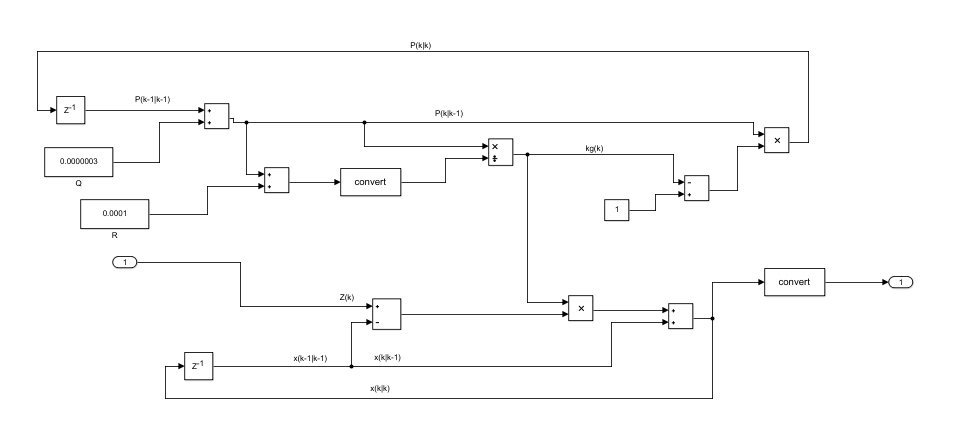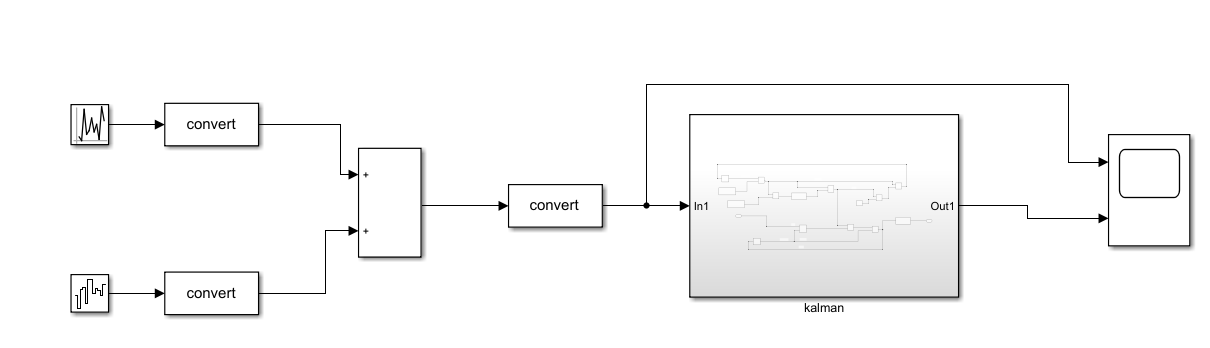具体设置kalman模块的Hdl Coder参数模式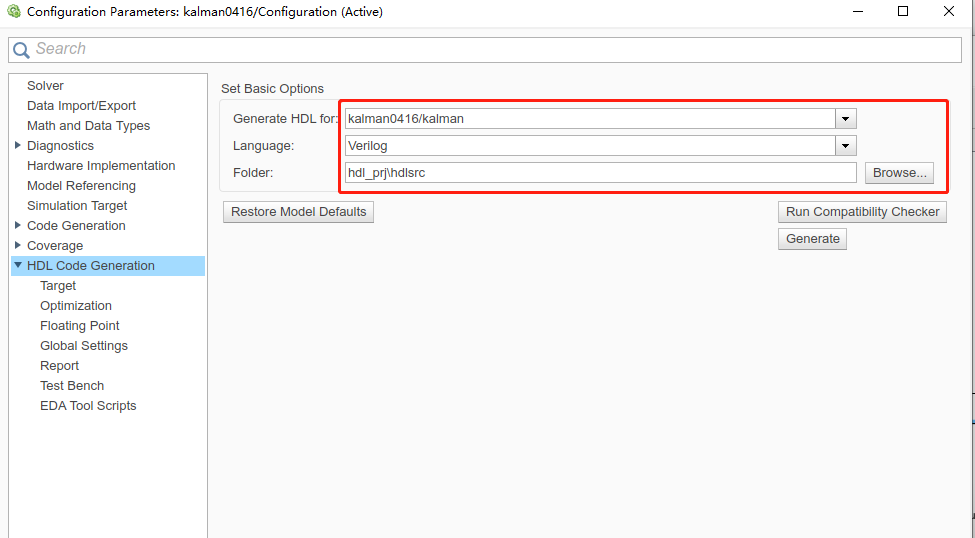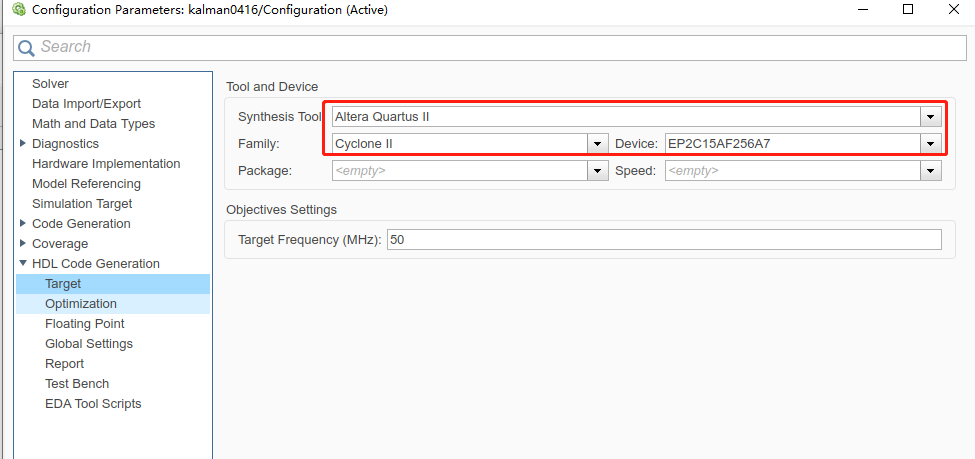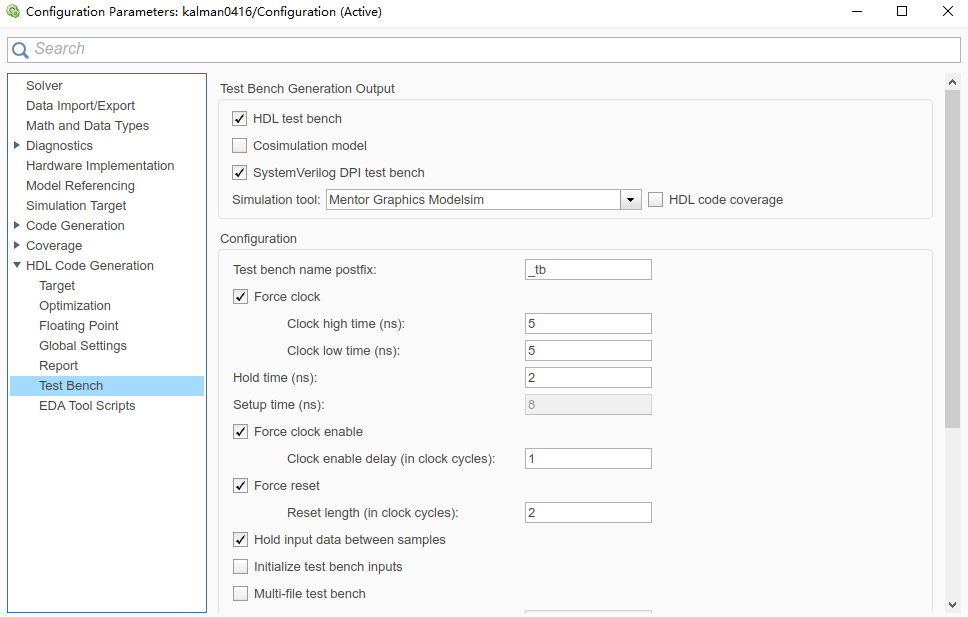最后点击“Generate HDL”与“Generate Test Bench”，生成verilog源程序与测试代码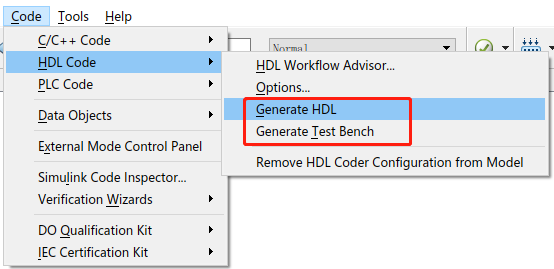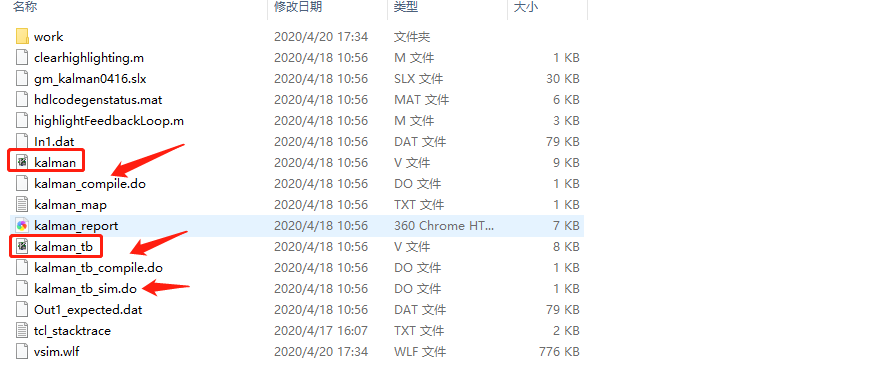1）Q=0.003，R=0.0001，，预计结果滤波后的噪声跟原始噪声相差不大。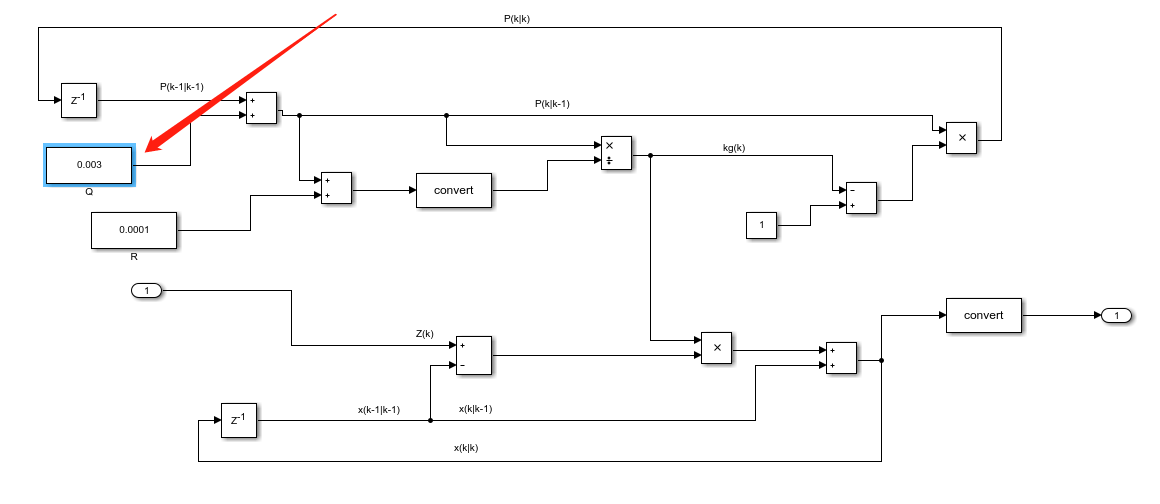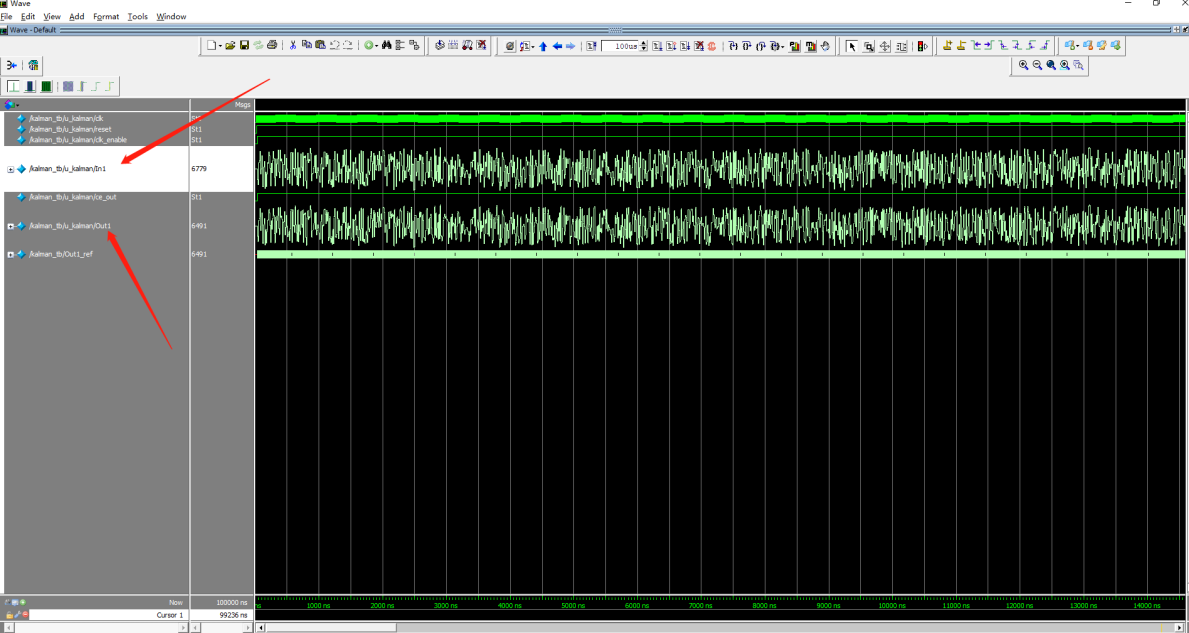2）Q=0.0000003，R=0.0001，预计滤波后的噪声跟原始噪声幅度值相差较大，滤波效果较好。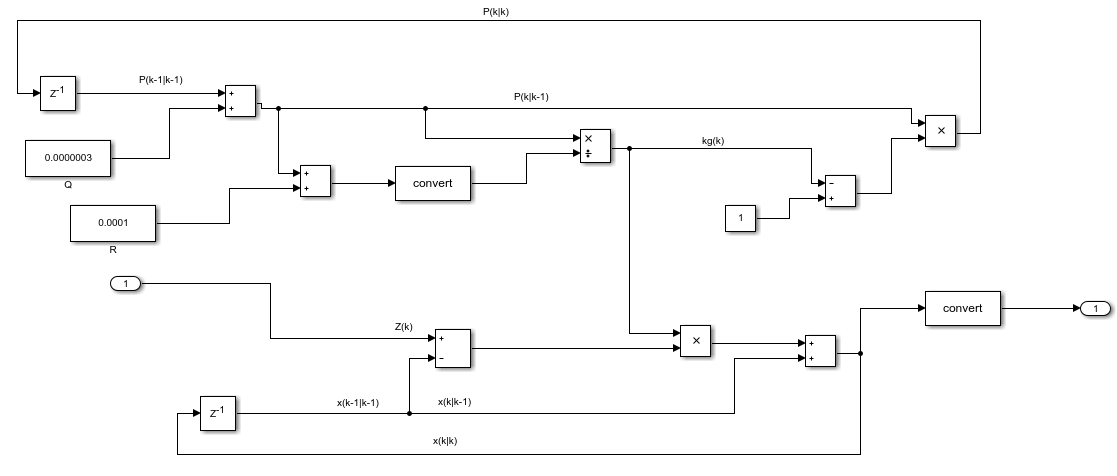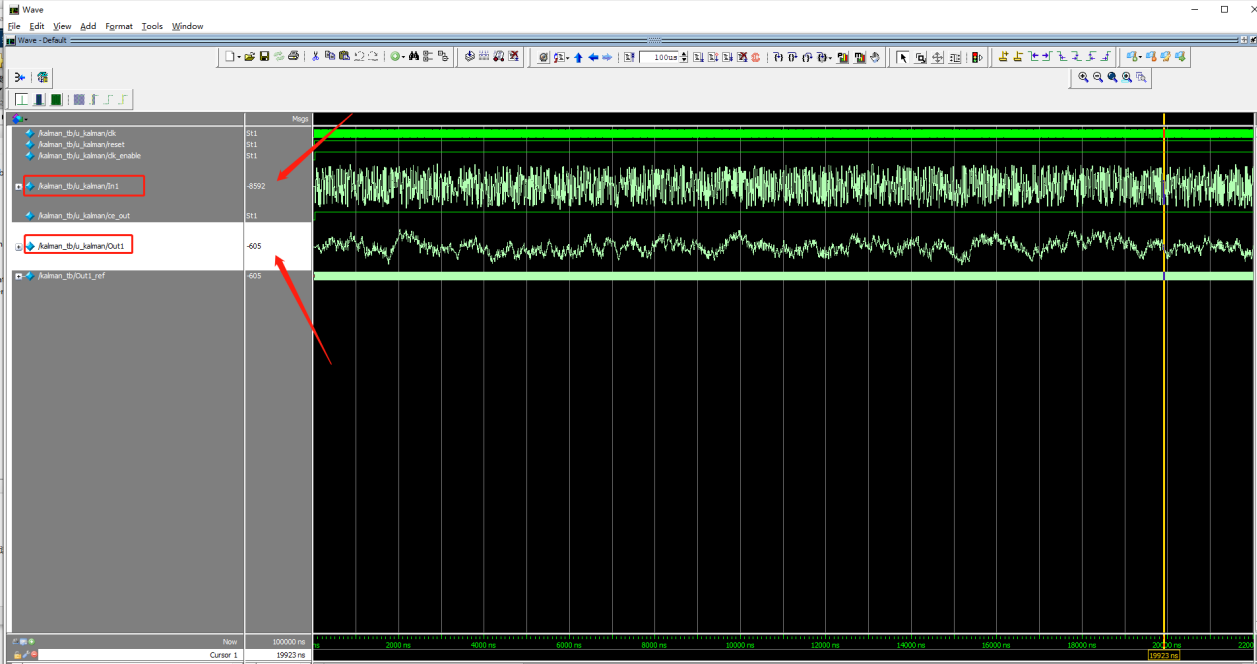Ps：最近一周心情太差，压力满满，茶饭不思，唯有将无限的精力投身于自己喜欢的FPGA身上了，希望早日摆脱雾霾，早日活力满满！